Yann LeCun wrote in a blog post:

If we show just a few drawings of cows to small children, they’ll eventually be able to recognize any cow they see.

Humans can learn to drive a car in approximately 20 hours with very little supervision.

On the other hand, completely autonomous driving confuses our greatest ML systems trained thousands of hours.

That’s because people rely on prior knowledge of how the world works. This prior knowledge is what is known as common sense.

In this context self-supervised learning is simple a way to learn the common sense.

Both self-supervised and semi-supervised learning are currently hot trends in machine learning.

For instance the rise of non-supervised learning in Word2Vec and GloVE somehow brought BERT as a self-supervised learning model which addresses the problems Word2Vec and GloVE have.

Word2Vec will generate the same vector for each word from the dictionary. The problem is then when we use homonyms or multiple-meaning words.

BERT will generate different vectors for the same word being used in two different contexts, so it fixes the problems with homonyms.

GloVe is very similar to Word2Vec and one may perform better than other just based on a dataset of choice.

Today, the NLP trend is either self-supervised or semi-supervised if we consider the dominant models such as: RoBERTa, XLM-R, GPT-2, GPT-3, T5, etc.

## What is self-supervised learning?

In self-supervised learning labels are generated from the data itself.

If we have a sentence: This is a cat.

We can label each word with a specific word type, or we can label the whole sentence either positive or negative. This is a supervised approach.

Self-supervised learning approach is what BERT will do. If we omit the word and try to guess it.

This is [mask] cat.

Now, the label is whatever is missing, and the task of self-supervised machine learning is to figure out the label given the masked sentence.

We call it self-supervised learning because we create the labels from the data, otherwise it would be unsupervised learning.

To create the labels in the previous case we used the technique called masking.

Don’t confuse self-supervised learning with semi-supervised learning which is a combination of supervised and unsupervised machine learning methods. In semi-supervised learning some examples are labeled and the others are unlabeled.

## Predicting hidden parts from non hidden parts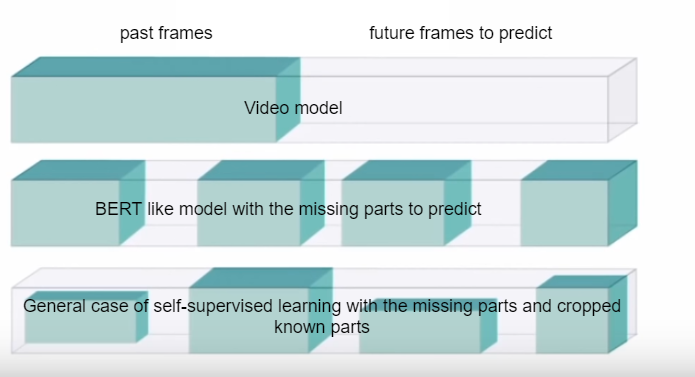There are some obvious differences to notice when applying self-supervised learning on text and on video.

For the text problems you can do masking which is a classification problem at the end. The masked words can be anything from the vocabulary. The dimensionality of the classification problem is the size of the vocabulary.

In case of the vision problems we don’t have a discrete problem to solve. If we mask a part of the image and try to reconstruct what’s missing there will be many ways to replace what’s missing.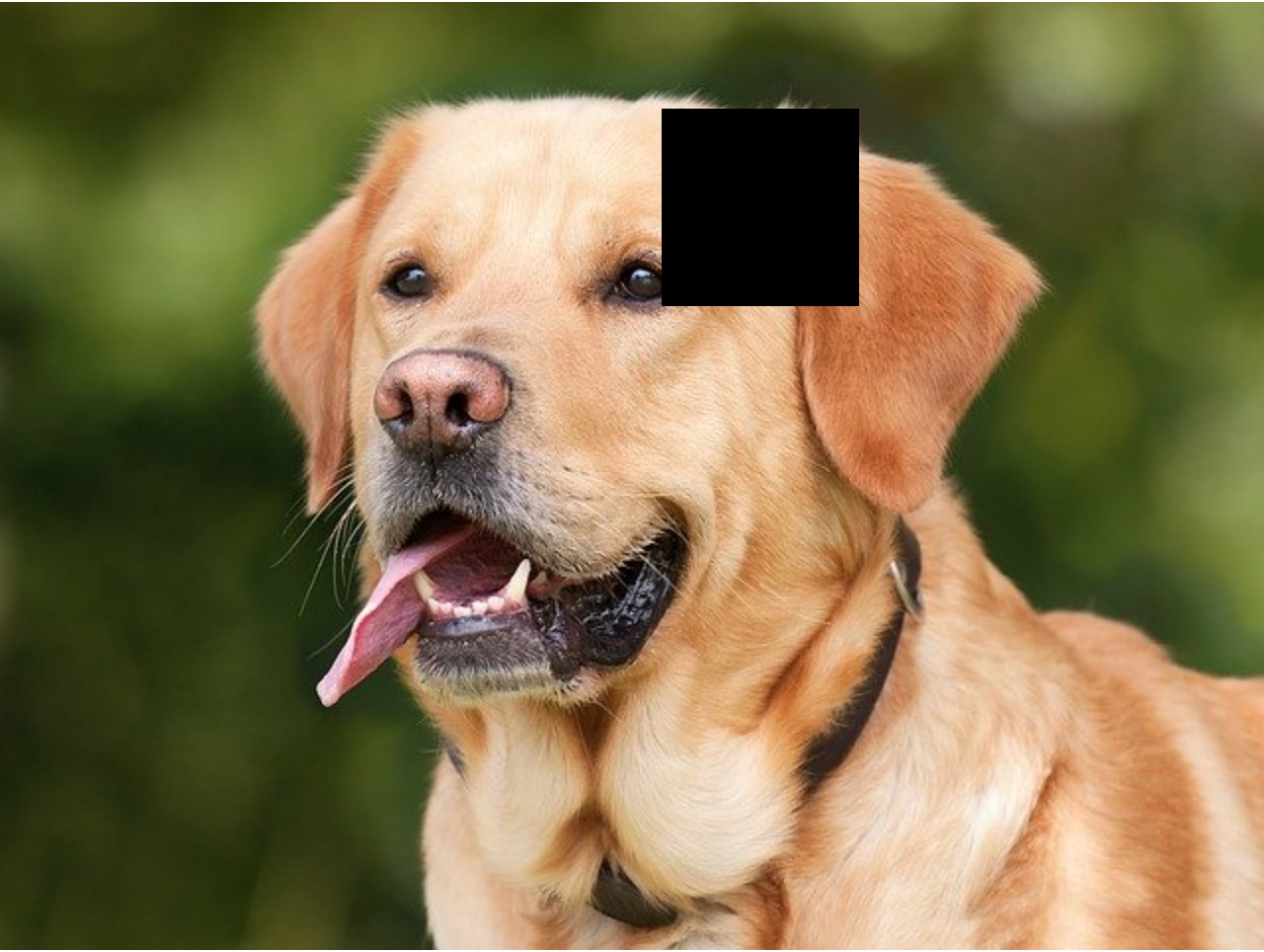From this reason it is hard to reason about the problem dimensionality in case of vision tasks.

## Introducing the Energy function

In computer vision a loss function can also be called the energy function.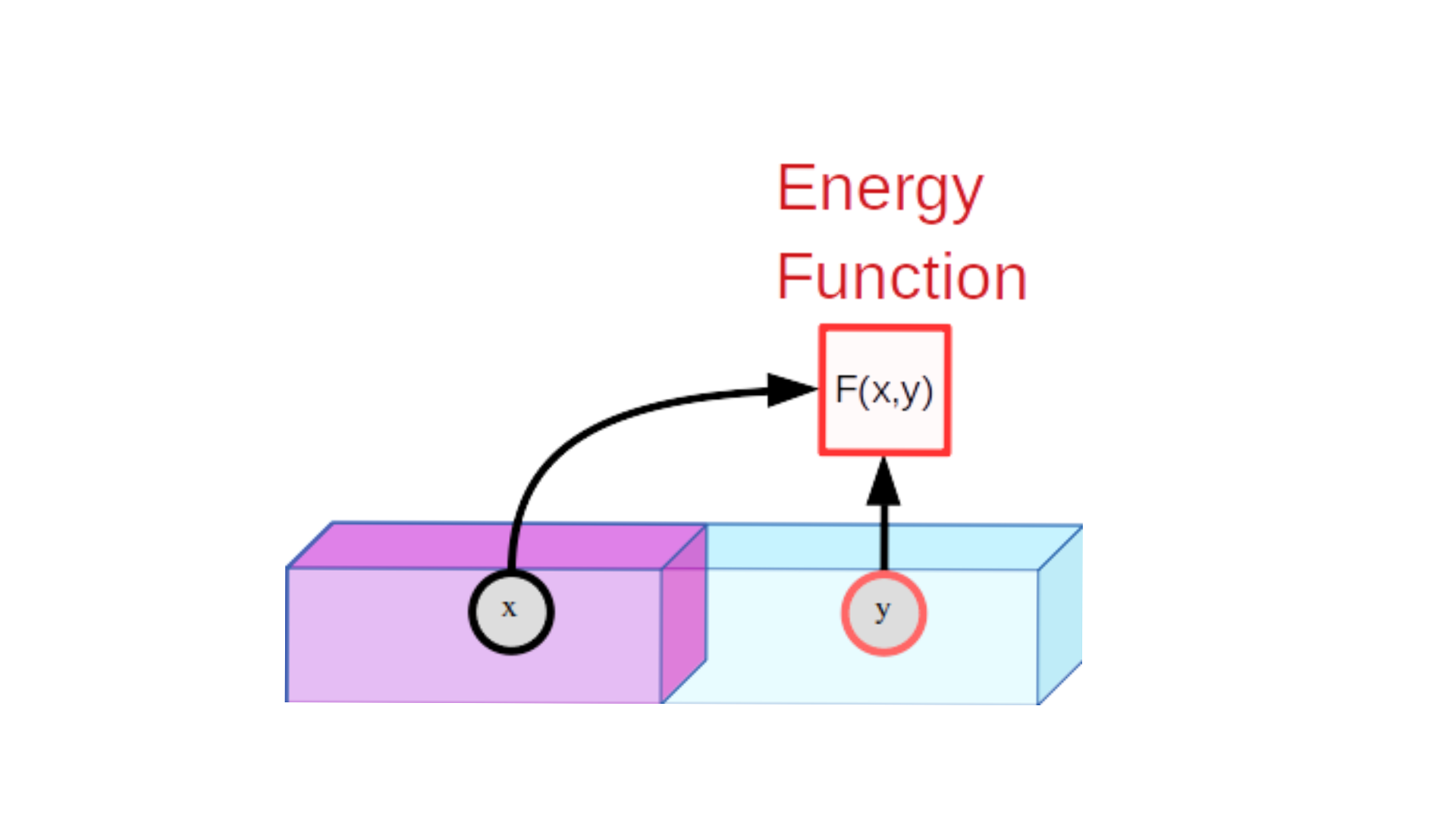We can reason about $y$ as a continuation of $x$ based on $F(x,y)$. If $F(x,y)$ is low the continuation is quite possible, else it isn’t.

## SSL by comparing image features

Probably the most popular task for self-supervised learning are siamese images. You can create them with slight distortion of the original such as the crop effect.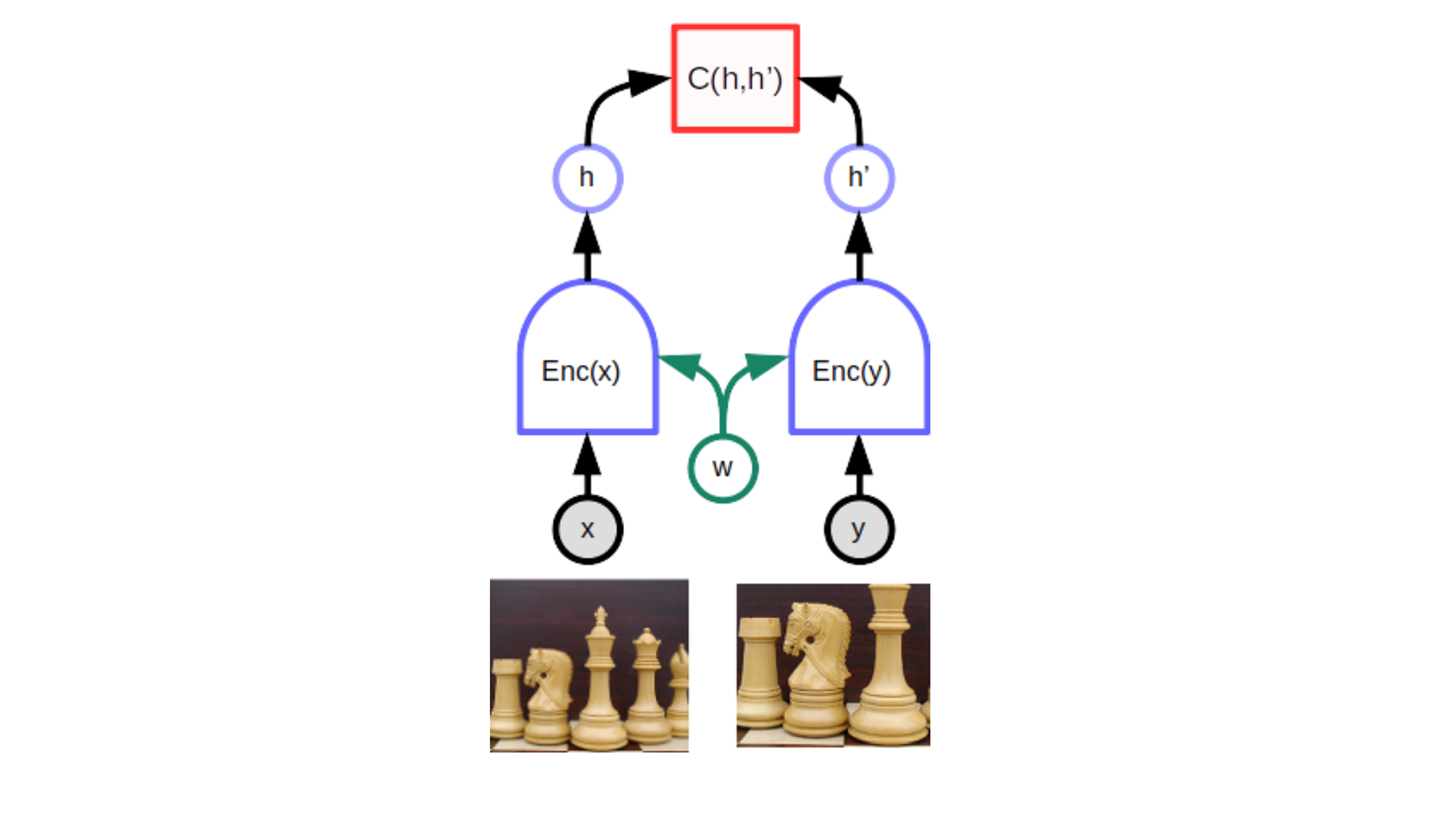This model is called the joint embedding architecture. It has two encoders creating hidden representations $h$. Encoders share the same weights. The cost function or energy function is based on the inner (dot or scalar) product between hidden representations.

The inner product is bigger if the features are close enough, else it’s smaller.

Here we are comparing the image features $h$. This is different from comparing the images directly.

### The collapse problem with siamese images

There is a catch with these siamese images. If we had just two similar images we would run to a problem called collapse.

We avoid collapsing by introducing contrastive images to the original chess pieces. Contrastive image means somehow different from the original.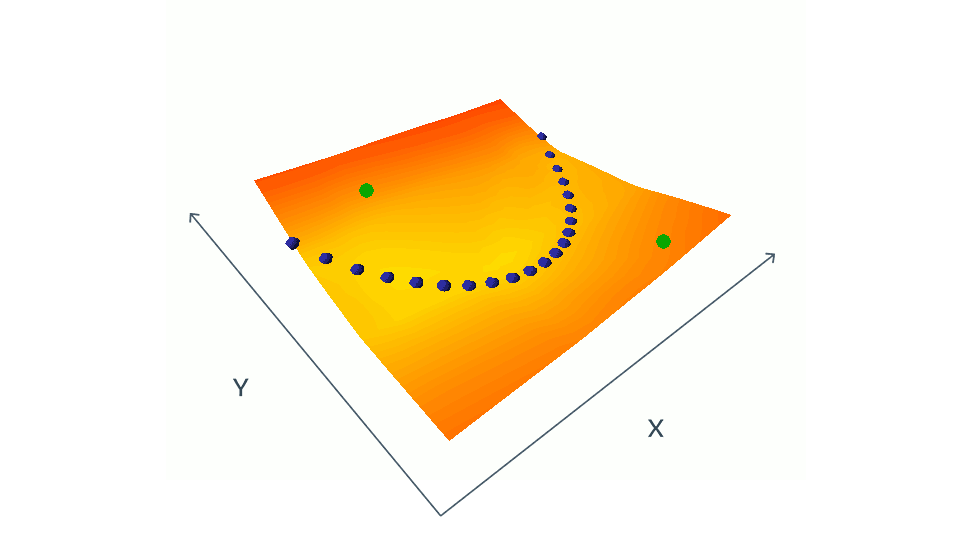The blue dots from the animation are similar images and green dots are contrastive images.

Yann explains in the article the reasoning behind introducing the contrastive images:

When $x$ and $y$ are slightly different the system is trained to produce the low energy. The difficult part is to train the model so it produces high energy.

This means we need to create different embeddings (features) for different images in order for the model to effectively learn.

## Latent-variable predictive architecture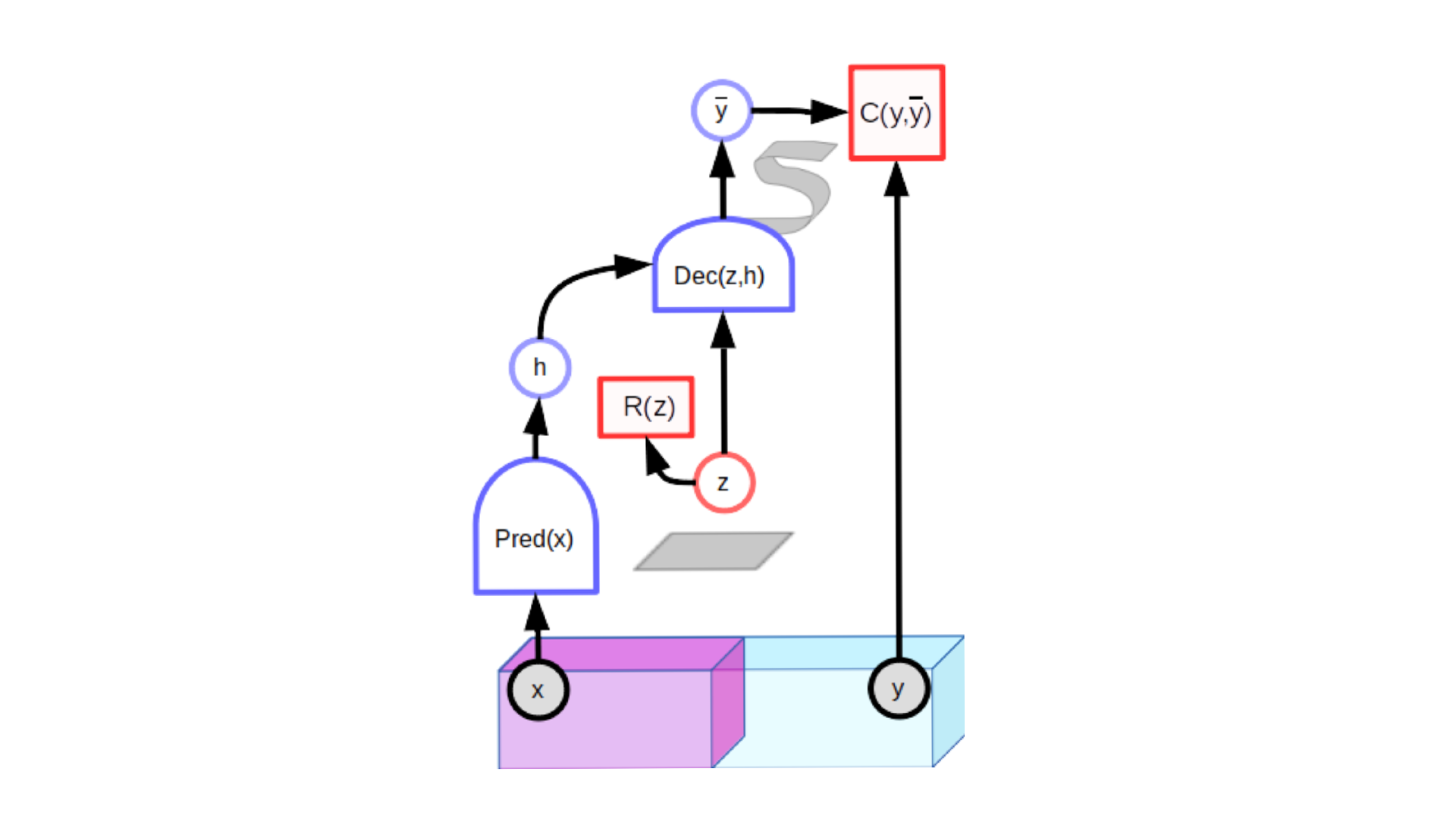The second method to avoid collapse problems is regularization of the latent variable in a specific architecture called latent-variable predictive architecture.

In the image hidden representation $h$ is the output of the encoder from the original $x$. The encoded features $h$ would convert to a single prediction after the decoder part if there would be no latent variable.

By picking different latent variables $z$ from the latent space we enforce different possible predictions (not just one).

The skill to balance the impact of the input image $x$ and latent variable $z$ is crucial when creating a good model.

If the impact of input $x$ is very small we get the typical GAN model.

If the impact of the latent variable $z$ is very small we have the basic predictive model.

The gain with latent-variable predictive architecture is that we don’t need to use contrastive images. We can learn just from similar images.

## Conclusion

Self-supervised learning is the latest trend in machine learning. Just to name GPT-3 and BERT models that use it.

BERT is an example of self-supervised learning for NLP tasks.

For visual tasks it is not possible to use classification like BERT does.

For visual tasks predictive models predicting a single output are limited.

Siamese networks deal with image features rather than comparing images itself. Siamese networks introduce contrastive learning techniques to eliminate the collapse problem.

Another approach is to use latent-variable predictive models. This is probably the way to go for the visual self-supervised tasks.

Latent-variable predictive models:

• eliminate the need for contrastive images because we may need a lot of contrastive images to train
• immediately introduce multiple outputs based on the latent variable $z$.